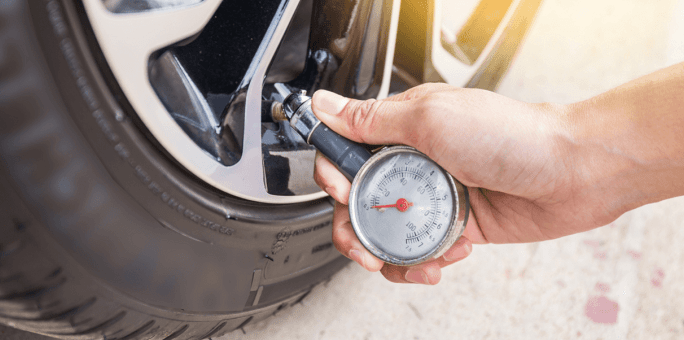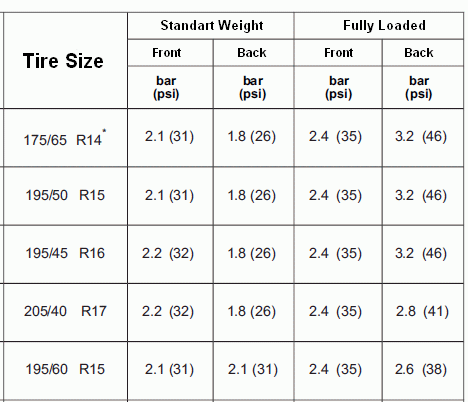# What You Didn't Know About Tire Pressure

We must check the tire pressure of our vehicle periodically. No driver wants to encounter an unexpected tire problem in traffic. Especially drivers who will take the long journey. In this article, we will find answers to questions such as; "What is Tire Pressure?", "What is the Ideal Tire Pressure for Long Distance?" and "How to Find the Recommended Vehicle Tire Pressure?”.What is Tire Pressure?

Tire pressure can be defined as the gas density between the outer surface of the vehicle wheel and the vehicle tire. It is very important that we use our vehicle at the correct tire pressure. So, what can be said if we come to the benefits of correct tire pressure;

• It increases both the performance and safety of the vehicle.
• It prevents the rim from coming off the wheel in turns and increases the durability of the tire.
• It increases the vehicle's handling and steering control.
• At the same time, using the right tire pressure will make you save fuel.What is the Ideal Tire Pressure for Long Distance?

One of the factors we should check before taking a long journey is tire pressure. Tire pressure needs to be at the correct PSI or Bar value. If there is something strange in these values, it may be best not to take the vehicle on the road. The correct tire pressure values ​​are usually given in your vehicle's owner's manual. You can reach the value you want with a simple math. 1 bar equals 14.50 psi. If your recommended vehicle tire pressure is 2.2 bar, multiply it by 14.50 to find the correct psi value. In this case, the recommended tire pressure in the given example will be 32 psi.

Vehicle tire pressure varies according to vehicle weight, load distribution and tire dimensions. Let's examine what the tire air pressure should be according to the load distribution of the tire sizes outside the table above.

Tire Pressure of 195 55 R16

If the weight balance of the vehicle is equal;

• Two passengers traveling at the front, if the rear is empty, the front wheels are 34 psi, the rear wheels are 32 psi,

• If two passengers are traveling in the front and rear, the front wheels are 34 psi, the rear wheels are 34 psi,

• If two passengers in the front and 3 passengers in the rear are traveling, the front wheels should be 34 psi and the rear wheels should be 32 psi.

If the weight balance of the vehicle is at the front;

• Two passengers traveling at the front, if the rear is empty, the front wheels are 34 psi, the rear wheels are 32 psi,

• If two passengers are traveling in the front and rear, the front wheels are 36 psi, the rear wheels are 34 psi,

• If two passengers are traveling in the front and 3 passengers in the rear, the front wheels should be 36 psi and the rear wheels should be 36 psi.

Tire Pressure of 195 65 R15

If the weight balance of the vehicle is equal;

• Two passengers traveling at the front, if the rear is empty, the front wheels are 34 psi, the rear wheels are 32 psi,

• If two passengers are traveling in the front and rear, the front wheels are 34 psi, the rear wheels are 34 psi,

• If two passengers in the front and 3 passengers in the rear are traveling, the front wheels should be 34 psi and the rear wheels should be 36 psi.

If the weight balance of the vehicle is at the front;

• Two passengers traveling at the front, if the rear is empty, the front wheels are 34 psi, the rear wheels are 32 psi,

• If two passengers are traveling in the front and rear, the front wheels are 36 psi, the rear wheels are 34 psi,

• If two passengers in the front and 3 passengers in the rear are traveling, the front wheels should be 34 psi and the rear wheels should be 34 psi.

Tire Pressure of 205 55 R16

If the weight balance of the vehicle is equal;

• Two passengers traveling at the front, if the rear is empty, the front wheels are 36 psi, the rear wheels are 34 psi,

• If two passengers are traveling at the front and rear, the front wheels are 36 psi, the rear wheels are 36 psi,

• If two passengers in the front and 3 passengers in the rear are traveling, the front wheels should be 36 psi and the rear wheels should be 38 psi.

If the weight balance of the vehicle is at the front;

• Two passengers traveling at the front, if the rear is empty, the front wheels are 36 psi, the rear wheels are 34 psi,

• If two passengers are traveling at the front and rear, the front wheels are 38 psi, the rear wheels are 36 psi,

• If two passengers in the front and 3 passengers in the rear are traveling, the front wheels should be 38 psi and the rear wheels should be 38 psi.

Tire Pressure of 225 45 R17

If the weight balance of the vehicle is equal;

• Two passengers traveling in the front, if the rear is empty, the front wheels are 38 psi, the rear wheels are 36 psi,

• If two passengers are traveling at the front and rear, the front wheels are 38 psi, the rear wheels are 38 psi,

• If two passengers in the front and 3 passengers in the rear are traveling, the front wheels should be 38 psi and the rear wheels should be 40 psi.

If the weight balance of the vehicle is at the front;

• Two passengers traveling in the front, if the rear is empty, the front wheels are 38 psi, the rear wheels are 36 psi,

• If two passengers are traveling at the front and rear, the front wheels are 40 psi, the rear wheels are 38 psi,

• If two passengers in the front and 3 passengers in the rear are traveling, the front wheels should be 40 psi and the rear wheels should be 40 psi.

Tire Pressure of 225 40 R18

If the weight balance of the vehicle is equal;

• Two passengers traveling in the front, if the rear is empty, the front wheels are 40 psi, the rear wheels are 38 psi,

• If two passengers are traveling at the front and rear, the front wheels are 40 psi, the rear wheels are 40 psi,

• If two passengers in the front and 3 passengers in the rear are traveling, the front wheels should be 40 psi and the rear wheels should be 42 psi.

How to Find the Recommended Vehicle Tire Pressure?

We can learn the recommended tire pressure values in several ways such as;

• Vehicle manuals

• On the information sticker on the driver's door or fuel tank cap